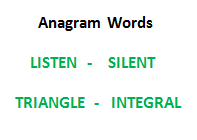# Check whether two strings are anagrams of each other using unordered_map in C++

Write a function to check whether two given strings are an Anagram of each other or not.

An anagram of a string is another string that contains the same characters, only the order of characters can be different.

For example, “abcd” and “dabc” are an Anagram of each other.## Recommended: Please try your approach on {IDE} first, before moving on to the solution.

Approach: Unordered Map can also be used to find if any two given strings are anagrams or not. The idea is to store each character of the first string in the map, with its frequency as value, and after that check for each character of the second string in the map, and if the character is found in the map, reduce its frequency value from the map. If the frequency of a character becomes 0, erase it from the map and at last if the map becomes empty, that means all the characters of the first string are there in the second string with the same number of occurrences(frequency of each character).

Implementation:

 `// C++ program to check whether ` `// two strings are anagrams of ` `// each other or not, using Hashmap ` ` `  `#include ` `#include ` `using` `namespace` `std; ` ` `  `// Function to check whether two strings ` `// are an anagram of each other ` `bool` `isanagram(string s1, string s2) ` `{ ` `    ``int` `l1 = s1.length(); ` `    ``int` `l2 = s2.length(); ` ` `  `    ``unordered_map<``char``, ``int``> m; ` `    ``if` `(l1 != l2) { ` `        ``return` `false``; ` `    ``} ` `    ``for` `(``int` `i = 0; i < l1; i++) { ` `        ``m[s1[i]]++; ` `    ``} ` ` `  `    ``for` `(``int` `i = 0; i < l2; i++) { ` `        ``if` `(m.find(s2[i]) == m.end()) { ` `            ``return` `false``; ` `        ``} ` `        ``else` `{ ` `            ``m[s2[i]]--; ` `            ``if` `(m[s2[i]] == 0) { ` `                ``m.erase(s2[i]); ` `            ``} ` `        ``} ` `    ``} ` `    ``return` `m.size() == 0; ` `} ` ` `  `// Test function ` `void` `test(string str1, string str2) ` `{ ` ` `  `    ``cout << ``"Strings to be checked:\n"` `         ``<< str1 << ``"\n"` `         ``<< str2 << ``"\n"``; ` ` `  `    ``if` `(isanagram(str1, str2)) { ` `        ``cout << ``"The two strings are"` `             ``<< ``"anagram of each other\n"``; ` `    ``} ` `    ``else` `{ ` `        ``cout << ``"The two strings are not"` `             ``<< ``" anagram of each other\n"``; ` `    ``} ` `    ``cout << endl; ` `} ` ` `  `// Driver program ` `int` `main() ` `{ ` `    ``// Get the Strings ` `    ``string str1 = ``"geeksforgeeks"``; ` `    ``string str2 = ``"forgeeksgeeks"``; ` ` `  `    ``// Test the Strings ` `    ``test(str1, str2); ` ` `  `    ``// Get the Strings ` `    ``str1 = ``"geeksforgeeks"``; ` `    ``str2 = ``"geeks"``; ` ` `  `    ``// Test the Strings ` `    ``test(str1, str2); ` `    ``return` `0; ` `} `

Output:

```Strings to be checked:
geeksforgeeks
forgeeksgeeks
The two strings areanagram of each other

Strings to be checked:
geeksforgeeks
geeks
The two strings are not anagram of each other
```

Related articles:

Attention reader! Don’t stop learning now. Get hold of all the important DSA concepts with the DSA Self Paced Course at a student-friendly price and become industry ready.

My Personal Notes arrow_drop_upI am a Computer Science Student at JIIT-Noida I have worked for Sanfoundry previously and contributed more than 50 articles

If you like GeeksforGeeks and would like to contribute, you can also write an article using contribute.geeksforgeeks.org or mail your article to contribute@geeksforgeeks.org. See your article appearing on the GeeksforGeeks main page and help other Geeks.

Please Improve this article if you find anything incorrect by clicking on the "Improve Article" button below.

Article Tags :

2

Please write to us at contribute@geeksforgeeks.org to report any issue with the above content.